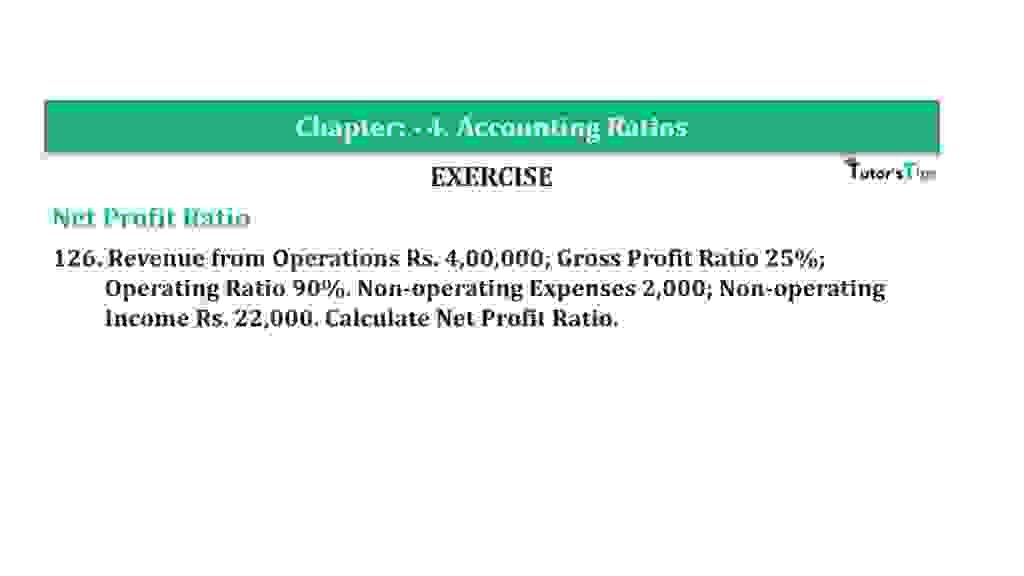# Question 126 Chapter 4 of +2-B – T.S. Grewal 12 ClassQuestion 126 Chapter 4 of +2-B

Net Profit Ratio

126. Revenue from Operations Rs. 4,00,000; Gross Profit Ratio 25%;
Operating Ratio 90%. Non-operating Expenses 2,000; Non-operating
Income Rs. 22,000. Calculate Net Profit Ratio.

### The solution of Question 126 Chapter 4 of +2-B: –

 Net Profit = Operating Profit + Non Operating Incomes -Non Operating Expenses = Rs. 40,000 + Rs. 22,000 – Rs. 2,000 = Rs. 60,000 Operating Profit Ratio = 100 −Operating Ratio = 100-90 =10% = Rs. 1,31,500 Operating Profit = Rs. 4,00,000 x 10% = Rs. 40,000

 Net Profit Ratio = Net Profit X 100 Net Sales
 Net Profit Ratio = Rs. 60,000 X 100 Rs. 4,00,000 = 15%

Balance Sheet: Meaning, Format & Examples

Thanks, Please Like and share with your friends

Comment if you have any question.

Also, Check out the solved question of previous Chapters: –

### Check out T.S. Grewal +2 Book 2020@ Official Website of Sultan Chand PublicationT.S. Grewal’s Analysis of Financial Statements